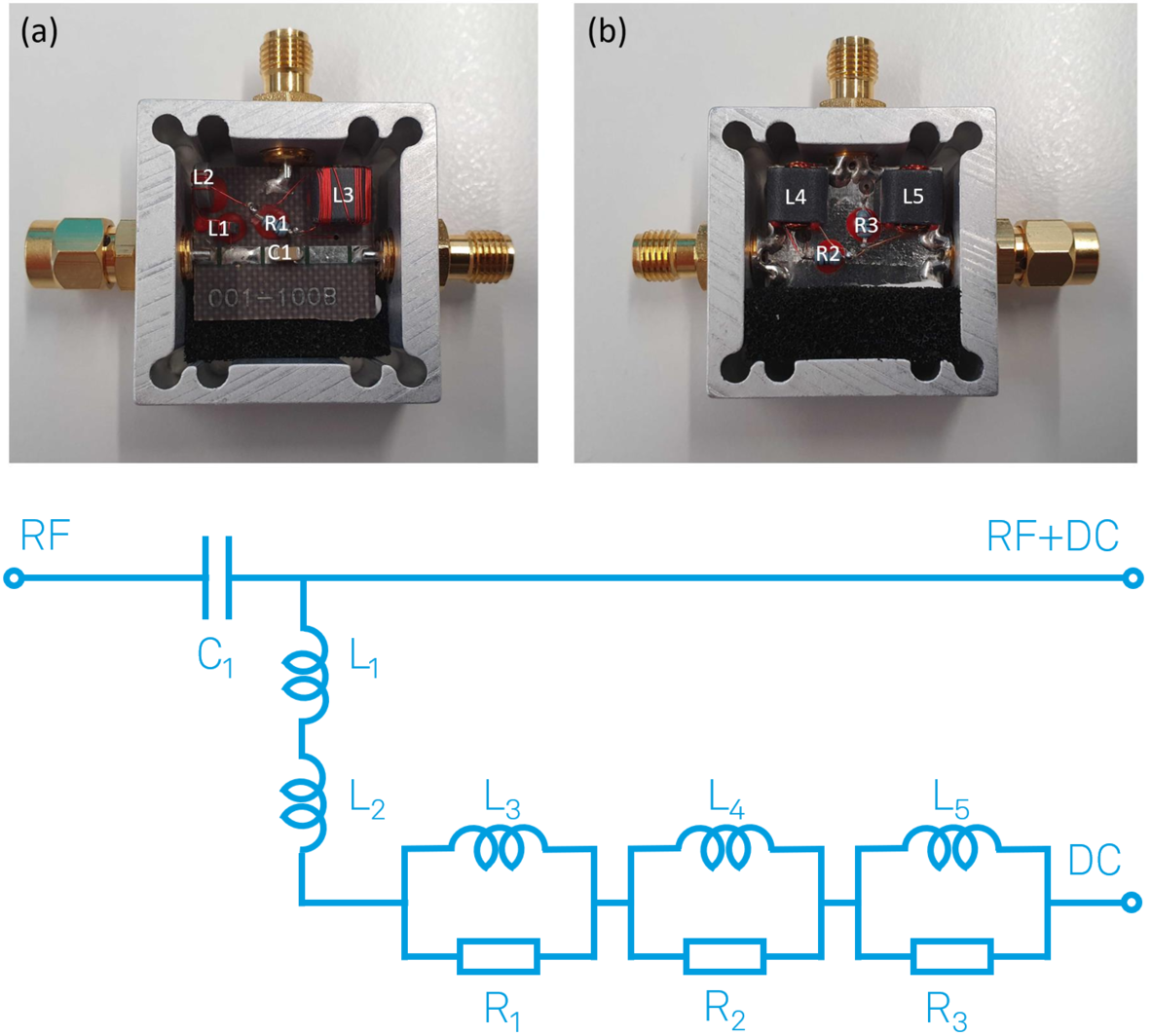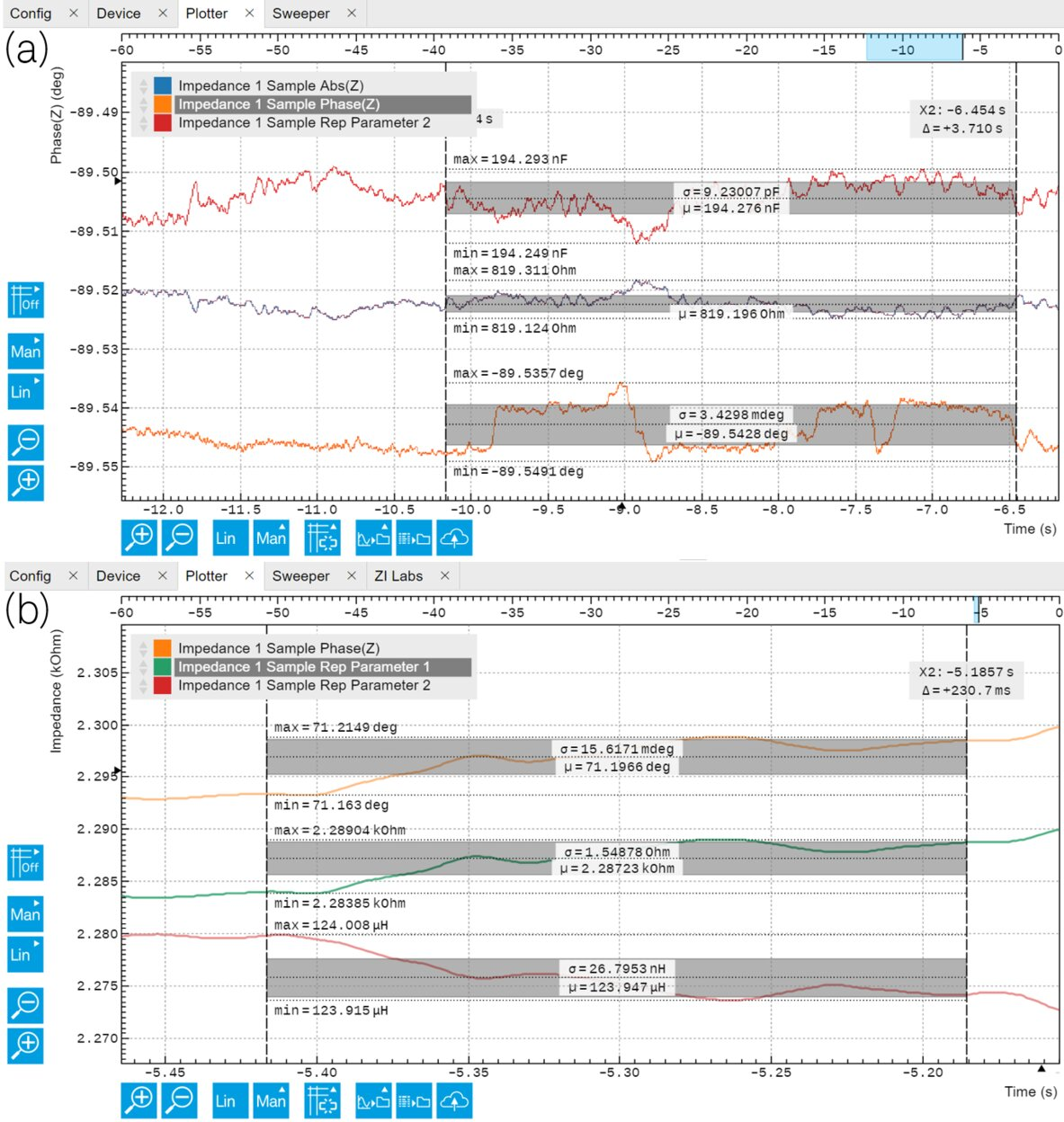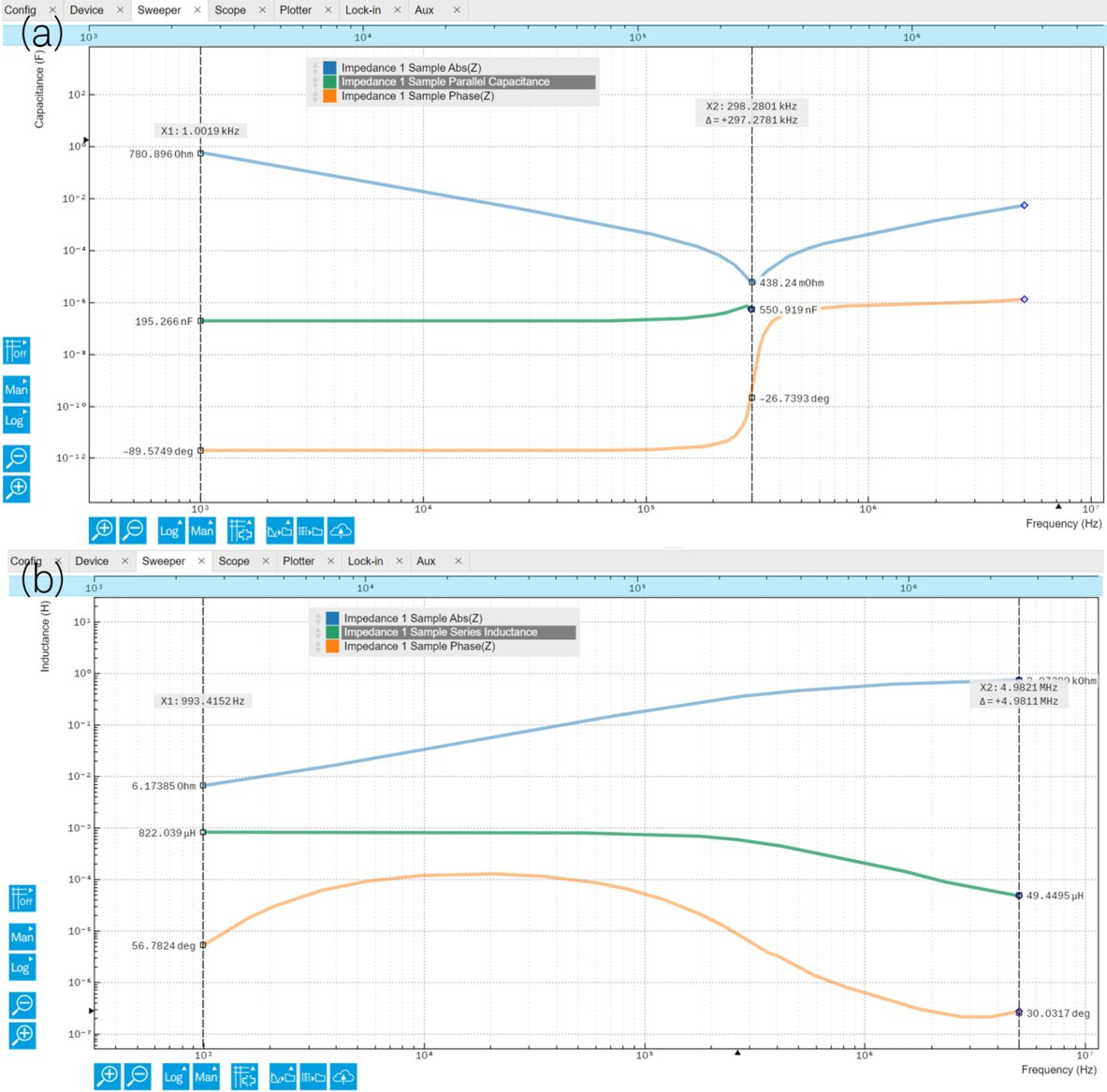# Impedance Characterization of a Wideband Bias Tee

April 28, 2022 by Meng Li

A bias tee is often used to add a DC offset to an RF signal ideally without affecting its transmission. In the simplest format, this is often achieved with an L||C circuit, where the high-frequency RF signal passes through the capacitor C, and the DC counterpart is injected across the inductor L.

However, a closer look at a commercial wideband bias tee can tell us a different story. In this blog post, we examine Mini-Circuits ZFBT-4R2GW+, a wideband bias tee from 0.1 MHz and 4200 MHz. As shown by Figure 1, this bias tee is composed of 1 capacitor (C1), 5 inductors (L1 to L5), and 3 resistors (R1 to R3). The equivalent circuit model of the bias tee can thus be built 'intuitively'.Figure 1: Top: teardown photos of a Mini-Circuits ZFBT-4R2GW+ bias tee. Bottom: an intuitive equivalent circuit of the bias tee.

We can begin the measurements by probing each (or each pair of) components inside the bias tee one by one. Hopefully, these measurements can hint us some useful parameters to further analyze the circuit model. Two measurement examples are shown in Figure 2, which were made with flying probes attached to an MFITF carrier card inserted into the MFITF. The MFITF impedance test fixture was mounted to the MFIA impedance analyzer directly on the front panel. Other probes can also be connected directly to the BNC connectors to take these measurements.

In the case of C1, we measure at a fixed frequency of 1 kHz using the LabOne Plotter module. For the L3||R1 pair, we measure instead at 1 MHz. These frequencies are arbitrarily chosen, however, it is important to see that none of these components are ideal. The parasitic resistance of C1, for instance, can contribute to the insertion loss of the RF line.Figure 2: LabOne screenshots showing the impedance of (a)C1 at 1 kHz and (b)L3||R1 at 1 MHz. Parasitic impedances can be clearly seen.

We can investigate further with the help of LabOne Sweeper module. Here we measure the impedance between the RF and RF+DC ports (high-f path), or between DC and RF+DC ports (low-f path). Such an impedance can be understood as the transfer function between the two ports. It is more descriptive than the (power) insertion loss since the impedance is a vector and carries additional phase information.

For simplicity, we only sweep between 1 kHz and 5 MHz with 200 frequency points in total. The high-f path in Figure 3(a) shows that C1 has about 195 nF value at 1 kHz, but its self-resonation happens at just 305 kHz. Given that the bias tee is designed to operate between 100 kHz and 4.2 GHz, C1 is mostly used in its inductive region (Is it still appropriate to call it a capacitance?). The low-f path also illustrates that the total inductance also is not a constant, particularly at working frequencies above 100 kHz. In fact, it is even closer to resistance with a phase angle at 30 deg at 5 MHz. Despite so many non-idealities, it is worth noting the impedance of the low-f path is still about 100 times higher than the high-f path at this frequency. By Kirchhoff's law, we can easily interpret this bias tee as a current divider in 100:1 ratio. And that is how a low insertion loss is achieved.Figure 3: LabOne screenshots showing the impedance of (a)high-f path between RF and RF+DC ports and (b)low-f path between DC and RF+DC ports.

To characterize a commercial bias tee with the MFIA impedance Analyzer, we have first determined the nominal values of individual components inside, which allows us to create a model. But the subsequent measurements taken with the LabOne Sweeper Module show that the behavior of this bias tee deviates from the simplistic view based on the components visible in Figure 1, and allow us to conclude that it is a much more complicated device. Even an intuitive 9-element circuit model cannot fully describe its behavior. Introducing parasitic for each element into a more complete circuit may help, but would require significant effort to model. This shows the importance of measuring the impedance of such components, so that we can better understand their expected response in any circuits. Specifically, when using a bias tee in an impedance measuring circuit, in order to reach higher accuracy, careful user compensation would be required at all frequencies. This will be discussed in-depth in a future post.

The author would like to sincerely thank Johannes Herrmann for proposing the idea, conducting the measurements and simulation.